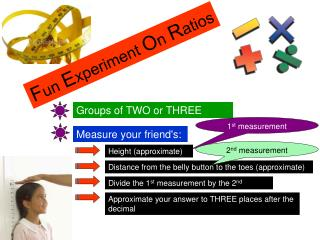DownloadDownload PresentationF un E xperiment O n R atios

# F un E xperiment O n R atios

Télécharger la présentation## F un E xperiment O n R atios

- - - - - - - - - - - - - - - - - - - - - - - - - - - E N D - - - - - - - - - - - - - - - - - - - - - - - - - - -
##### Presentation Transcript

1. Fun Experiment On Ratios Groups of TWO or THREE 1st measurement Measure your friend's: 2nd measurement Height (approximate) Distance from the belly button to the toes (approximate) Divide the 1st measurement by the 2nd Approximate your answer to THREE places after the decimal

2. Fun Experiment On Ratios The Ratio Should Be: 1.6180 …

3. Experiment !

4. The Fibonacci Series Leonardo of Pisa (1170-1250), nickname Fibonacci. He made many contributions to mathematics, but is best known of numbers that carries his name: 0, 1, 1, 2, 3, 5, 8, 13, 21, 34, 55, 89, 144, 233, 377, 610, 987, 1597, ... This sequence is constructed by choosing the first two numbers (the "seeds" of the sequence) then assigning the rest by the rule that each number be the sum of the two preceding numbers.

5. Take the RATIO of two successive numbers in Fibonacci's series, (1, 1, 2, 3, 5, 8, 13, ..) and divide each by the number before it. 1/1 = 1,   2/1 = 2,   3/2 = 1·5,   5/3 = ?,   8/5 = ?, 13/8 = ?, 21/13 = ? Use your calculator and plot a graph of these ratios and see if anything is happening. You'll have DISCOVERED a fundamental property of this RATIO when you find the limiting value of the new series!

6. The Golden Ratio Throughout history, the ratio for length to width of rectangles of 1.61803 39887 49894 84820 has been considered the most pleasing to the eye. This ratio was named the golden ratio by the Greeks. In the world of mathematics, the numeric value is called "phi", named for the Greek sculptor Phidias. The space between the columns form golden rectangles. There are golden rectangles throughout this structure which is found in Athens, Greece.

7. Examples of art and architecture which have employed the golden rectangle. This first example of the Great Pyramid of Giza is believed to be 4,600 years old, which was long before the Greeks. Its dimensions are also based on the Golden Ratio.

8. Pythagorean Connection

10. Unpacking

11. Course 2 12 – 2 640 - 645

12. Course 3 3 – 5 162 - 166

13. Course 3 3 – 6 167 - 171

14. Course 3 3 – 7 173 - 178

15. Algebra 1

16. Algebra 1

17. Geometry

18. Pythagorean Connections

20. Pythagorean Connections

21. Very Interesting Very Interesting

22. 12 Equal sized Sticks Area 9 Area 5 Perimeter 12 Perimeter 12

23. The Challenge Objective: Area 4 Perimeter 12

24. Very Interesting I should agree I agree

25. THIRD GRADE Handout Booklet: Pages 1-2

26. THIRD GRADE Developing NUMBER SENSE Handout Booklet: Pages 3 Pages 4- in today’s handout provide a sampling of how Number Sense develops across the grade levels. Your task is to TEACH someone else about the MacMillan math program. List six key points you would include in your presentation.

27. THIRD GRADE Handout Booklet: Pages 4-

28. In Problem Solving Lessons Handout Booklet: Pages 3-4

29. Handout Booklet: Pages 1-2

30. Handout Booklet: Pages 9-